# Double Exponential Smoothing

This calculator provides a description and an example of the double exponential smoothing.

Simple exponential smoothing does not give 100% results. To get a clearer data using double exponential smoothing.

double-smoothing formula is based on the previous formula (simple smoothing), only now add another member, that changes the input the previous value (depending on the trend).

The formula: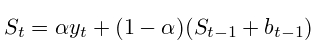where - it is a simple value [0; 1)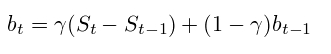where - [0, 1]

There are no one way to select the initial parameters, namely, S and b, data that affect the final result.

The methods that are used: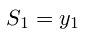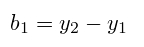b can still be calculated as follows:to predict the next value has a formula: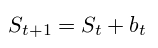to predict several meanings: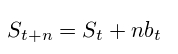This calculator can be changed and, as well as to compare the results with a simple smoothing.

The graph also shows the projected value of the following (smoothed average extended for 1 readout on the evidence).

﻿

Double exponential smoothing
A number dynamics
Import data
For separation fields can use one from these characters: Tab, ";" or ",":
Standard error double smoothing:
Standard error simple smoothing:
Double exponential smoothing: